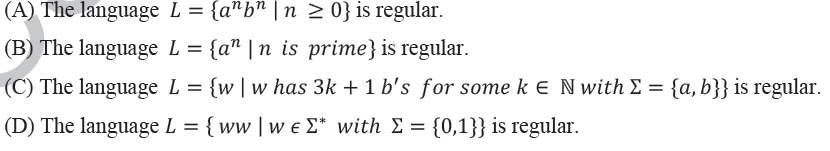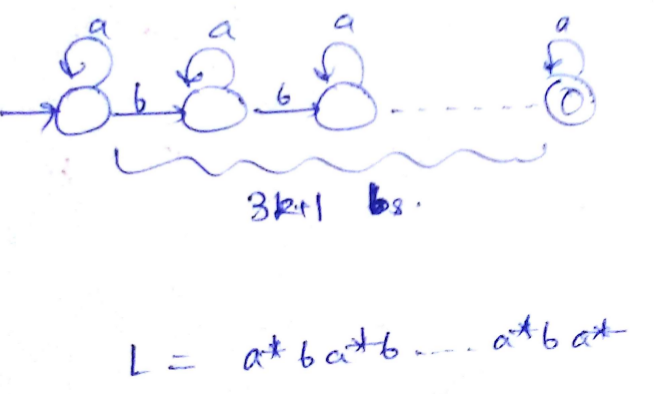# GATE | GATE-CS-2014-(Set-1) | Question 24

Which one of the following is TRUE?(A) A
(B) B
(C) C
(D) D

Explanation: (A) L = {a n b n |n >= 0} is not regular because there does not exists a finite automaton that can
derive this grammar. Intuitively, finite automaton has finite memory, hence it can’t track
number of as. It is a standard CFL though.

(B) L = {a n b n |n is prime} is again not regular because there is no way to remember/check if
current n is prime or not. Hence, no finite automaton exists to derive this grammar, thus it
is not regular.

(C) L = {w|w has 3k+1 bs} is a regular language because k is a fixed constant and we can easily
emulate L as a ∗ ba ∗ …..ba ∗ such that there are exactly 3k + 1 bs and a ∗ s surrounding each b in
the grammar.(D) L = {ww| w ∈ Σ ∗ } is again not a regular grammar, infact it is not even a CFG. There is no
way to remember and derive double word using finite automaton.

Hence, correct answer would be (C).
This solution is contributed by vineet purswani.

Quiz of this Question

My Personal Notes arrow_drop_up
Article Tags :

Be the First to upvote.

Please write to us at contribute@geeksforgeeks.org to report any issue with the above content.